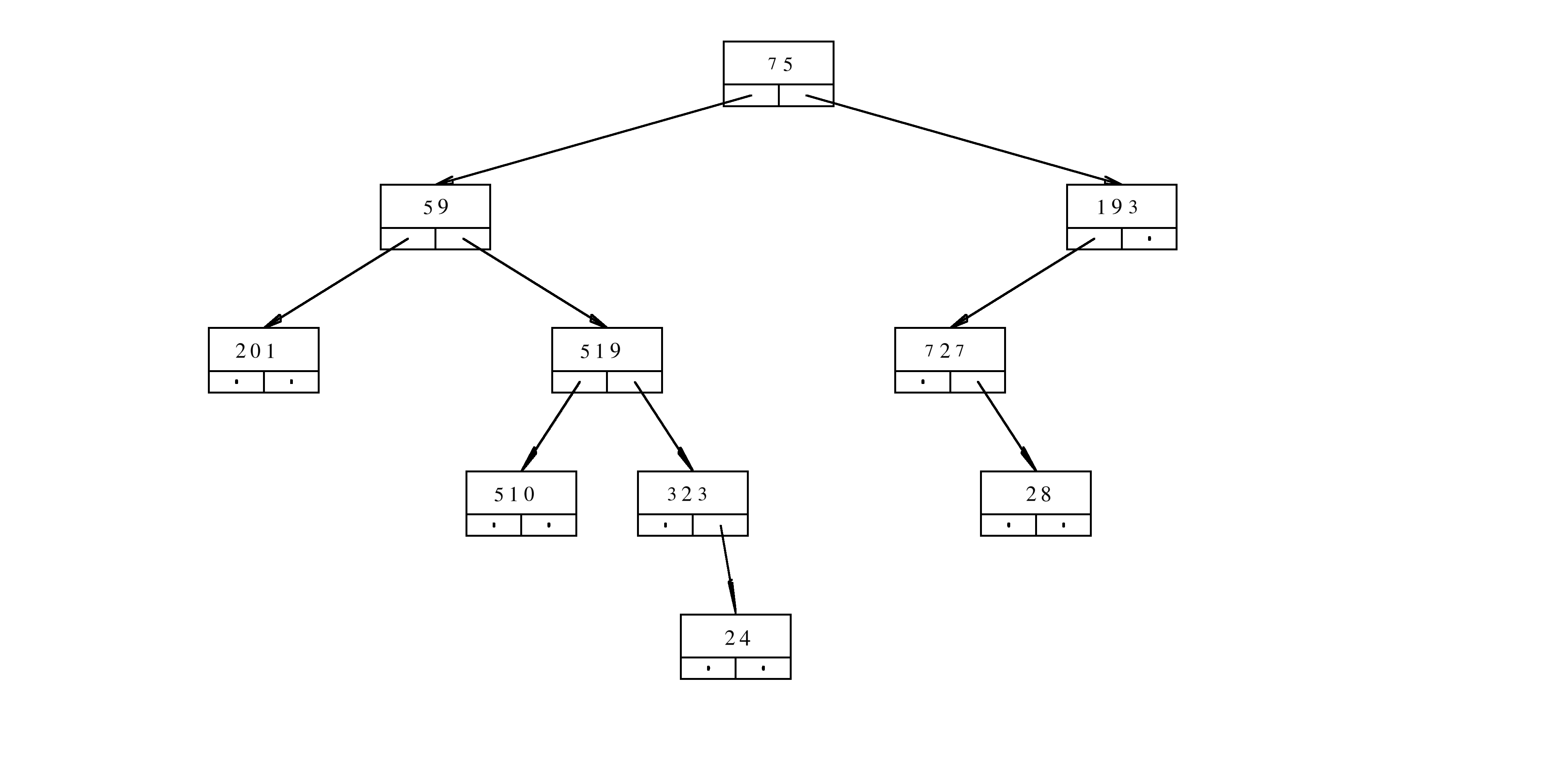MTH4300 Home

# MTH 4300: Final Practice 7

Problem 1.

Create program that generates the following sequence $$M$$ with a total of 10000 terms:

$M=\left(\underbrace{\underbrace{9,7,5,-2,3}_{5},\underbrace{9,7,5,-2,3}_{5}, \dots,\underbrace{9,7,5,-2,3}_{5}}_{10000}\right).$ Your code should replace // ??? so that the obtained function
int* generateSequence(){
// ???
}

creates the desired sequence when called from the main function with the command
int* M=generateSequence();


Problem 2.

What happens when the following program is executed? Provide a rigorous justification for your answer.

// myCode.cpp
// compile with
// c++ myCode.cpp -o myProgam -std=c++11
// execute with
// ./myProgram
#include<iostream>
class Mountain{
public:
int height;
Mountain();
Mountain(const Mountain & );
};
Mountain::Mountain(){
height=6;
}
Mountain::Mountain(const Mountain & c){
height=c.height+14;
}
int solve(Mountain x){
return x.height;
}
int get(Mountain & x){
return x.height;
}
int main(){
Mountain lassen;
std::cout<<2*solve(lassen) + 10*get(lassen)<<"\n";
return 0;
}


Problem 3.

Assume that cats and dogs are two objects of the type std::set<int> and that they satisfy \begin{eqnarray*}\mbox{cats} &=&\left\{17,18,19,20,21,22,23,24,25,26,27,28,29,30,31\right\},\newline \mbox{dogs}&=&\left\{29,30,31,32,33,34,35,36,37,38,39,40,41,42,43\right\}. \end{eqnarray*} What is printed on the standard output when the following block of code is executed?

for(int i=38;i<48;++i){
if(dogs.find(i)!=dogs.end()){
cats.insert(i);
}
}
std::cout<<cats.size()<<std::endl;


Problem 4.

How many times is the letter y printed on the standard output when the following program is executed? Provide a rigorous justification for your answer.

 // myProg.cpp
// compile with
// c++ myProg.cpp -o myP -std=c++11 -fno-elide-constructors
// execute with
// ./myP
#include<iostream>
class Queen{
public:
Queen();
Queen(const Queen&);
Queen(Queen&&);
void operator=(const Queen&);
void operator=(Queen&&);
~Queen();
};
Queen::Queen(){
std::cout<<"y";
}
Queen::Queen(const Queen& k){
std::cout<<"yy";
}
Queen::Queen(Queen&& k){
std::cout<<"yyy";
}
void Queen::operator=(const Queen& k){
std::cout<<"yy";
}
void Queen::operator=(Queen&& k){
std::cout<<"y";
}
Queen::~Queen(){
std::cout<<"y\n";
}
Queen slowFun(Queen& k){
Queen a=k;
return a;
}
int main(){
Queen k,b;
b=k;
for(int i=0;i<5;++i){
b=slowFun(k);
}
return 0;
}


Problem 5.

The node of the binary tree is defined in the following way

class TN{
public:
long content;
TN* aLeft;
TN* aRight;
};


The implementation of the function f is given below

long f(TN* a, long h){
if(a==nullptr){
return 1;
}
long c=a->content;
return (c%10)+(h%2)*f(a->aLeft,h+1)+(c%2)*f(a->aRight,h+1);
}


Assume that aRoot is the pointer to the root of the treeWhat is the result of the evaluation f(aRoot,3)? Provide a rigorous justification for your answer.

Problem 6.

The user input consists of a positive integer $$n$$ followed by a sequence of $$n$$ integers $$x_0$$, $$x_1$$, $$\dots$$, $$x_{n-1}$$. Create the program that calculates the total number of different outcomes that can be obtained as the results of the expressions $\pm x_0\pm x_1\pm x_2\pm\cdots\pm x_{n-1}$ for all choices of signs $$+$$ and $$-$$.

Example :

Input:
3
5 5 -5
Output:
4


Explanation: There are $$4$$ possible results. They are $$-15$$, $$-5$$, $$5$$, and $$15$$ and can be obtained in the following way: \begin{eqnarray*} -15&=&-x_0-x_1+x_2\newline -5&=& -x_0+x_1+x_2=+x_0-x_1+x_2=-x_0-x_1-x_2\newline 5&=&+x_0-x_1-x_2=-x_0+x_1-x_2=+x_0+x_1+x_2\newline 15&=&+x_0+x_1-x_2. \end{eqnarray*}